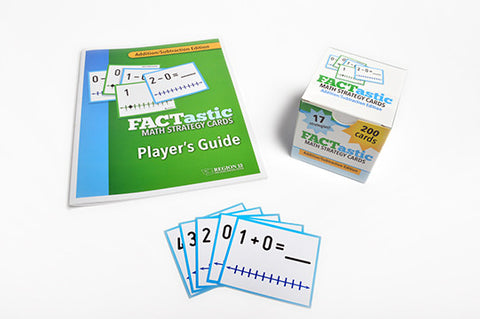## FACTastic Math Strategy System: Addition/Subtraction (Set of Cards and Spiral-Bound Teacher's Guide)

• \$ 4500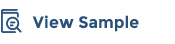FACTastic Math Strategy System: Addition/Subtraction makes the basic instruction of addition and subtraction for elementary-aged students more fun, while increasing true knowledge and fluency.

Our FACTastic Math Strategy System is a comprehensive program for teaching basic math facts through strategy-based instruction to build the flexible thinking and solid mathematical foundation that are critical for their success. The system includes:

• Model lessons that suggest how to teach each strategy through hands-on use of physical objects and visuals.
• Strategies and cards based on research that shows how concrete and visual representations can build students’ subject matter fluency.
• The FACTastic Teacher's Guide to walk teachers through the teaching of each strategy by emphasizing key points.
• Examples of think-aloud techniques in the Teacher's Guide.

##### ESF Alignment

Supports Lever 5.2 - Effective classroom routines and instructional strategies

##### Includes:
• FACTastic strategy cards (19 sets, 229 cards)
• Teacher's guide, spiral-bound (36 pages)
• FACTastic strategy cards instructional video series

FACTastic Math Strategy System was produced and is maintained in collaboration with Region 13's Special Education team and experts from the field.

We Also Recommend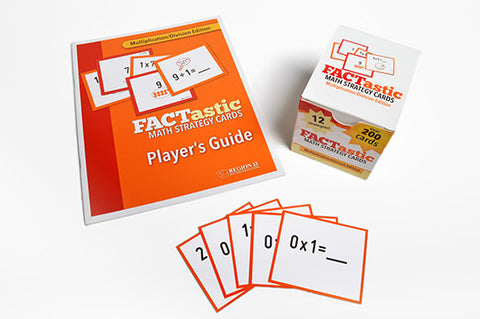FACTastic Math Strategy System: Multiplication/Division (Set of Cards and Spiral-Bound Teacher's Guide)

\$ 45.00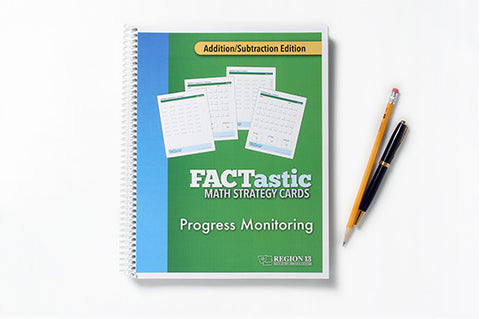FACTastic Math Progress Monitoring: Addition/Subtraction (Spiral-Bound)

\$ 20.00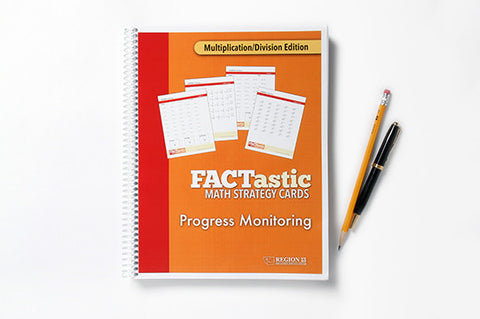FACTastic Math Progress Monitoring: Multiplication/Division (Spiral-Bound)

\$ 20.00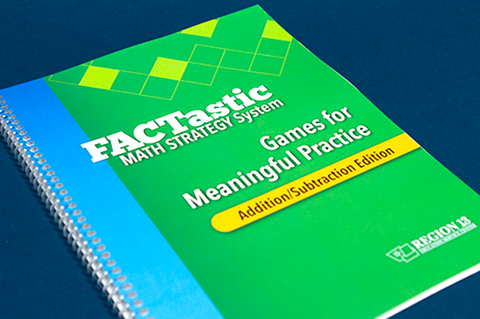FACTastic Math Strategy System Games for Meaningful Practice: Addition/Subtraction (Spiral-Bound)

\$ 30.00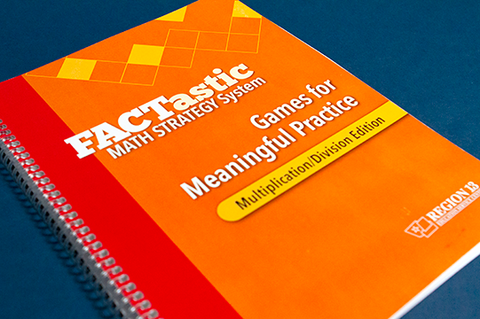FACTastic Math Strategy System Games for Meaningful Practice: Multiplication/Division (Spiral-Bound)

\$ 30.00
x